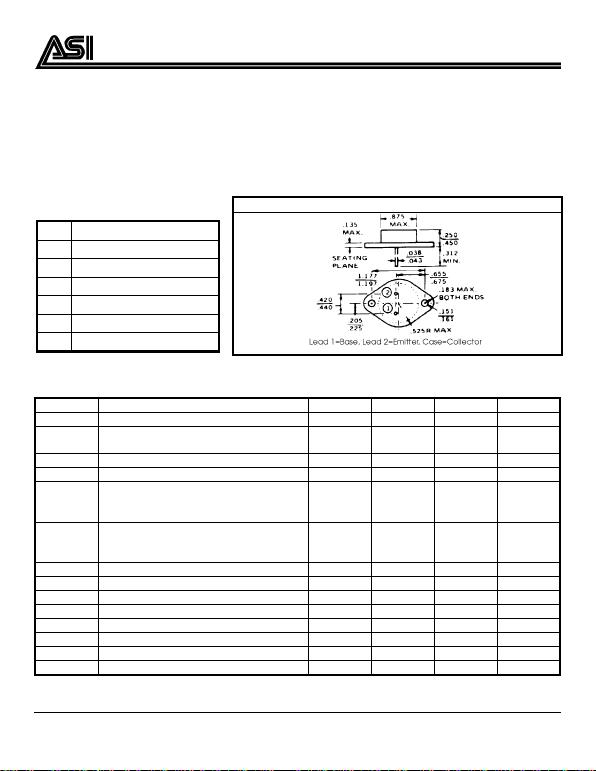 
 !
CHARACTERISTICS TC = 25 OCNONE
SY MBO L TES T CONDITI ONS MINIMUM TYP ICAL MAXIMUM UNITS
BVCEO IC = 200 m A 60 V
ICEV VCE = 100 V VBE = -1.5 V TC = 25 OC
TC = 150 OC5.0
30 mA
ICEO VCE = 30 V 700 µ
µµ
µA
IEBO VEB = 7. 0 V 5.0 mA
hFE
VCE = 2.0 V IC = 4. 0 A
VCE = 4.0 V IC = 4. 0 A
IC = 10 A
10
20
5.0
70
70 ---
VCE(SAT)
IC = 4.0 A IB = 0. 4 A
IC = 10 A IB = 3. 3 A
IC = 15 A IB = 7. 0 A
1.1
3.0
5.0
V
VBE(ON) VCE = 4.0 V IC = 4.0 A 0.7 1.8 V
Cob VCB = 10 V f = 1.0 M Hz 60 600 pF
ftVCE = 4.0 V IC = 1. 0 A f = 1.0 M Hz 0.8 6.0 MHz
Is/b VCE = 60 V t = 500 mS (NONREPETITIVE) 1.95 A
tdIC = 4. 0 A IB1 = IB2 = 0.4 A 0.5 µ
µµ
µS
trIC = 4.0 A IB1 = IB2 = 0.4 A 4.0 µ
µµ
µS
tsIC = 4. 0 A IB1 = IB2 = 0.4 A 3.0 µ
µµ
µS
tfIC = 4. 0 A IB1 = IB2 = 0.4 A 6.0 µ
µµ
µS
SILICO N NPN POW ER TRANSI STOR
2N3055A
DESCRIPTION:
The 2N3055A is Designed for
General Purpose Amplifier and
Switching Applications.
MAXIMUM RATINGS
IC15 A
IB7.0 A
VCE 60 V
PDISS 115 W @ TC = 25 OC
TJ-65 OC to +200 OC
TSTG -65 OC to +200 OC
θ
θθ
θJC 1.52 OC/W
PACKAGE STYLE TO 3